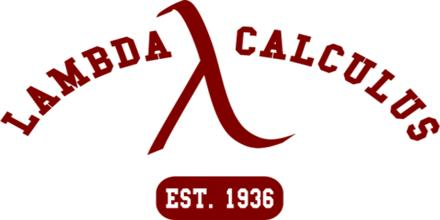Mathematic

# Lambda CalculusLambda calculus (also written as λ-calculus) is a formal system in mathematical logic and computer science for expressing computation by way of variable binding and substitution. It is important in programming language theory, and the symbol λ has even been adopted as an unofficial symbol for the field. Lambda Calculus consists of a language of lambda terms, which is defined by a certain formal syntax, and a set of transformation rules, which allow manipulation of the lambda terms. These transformation rules can be viewed as an equational theory or as an operational definition.